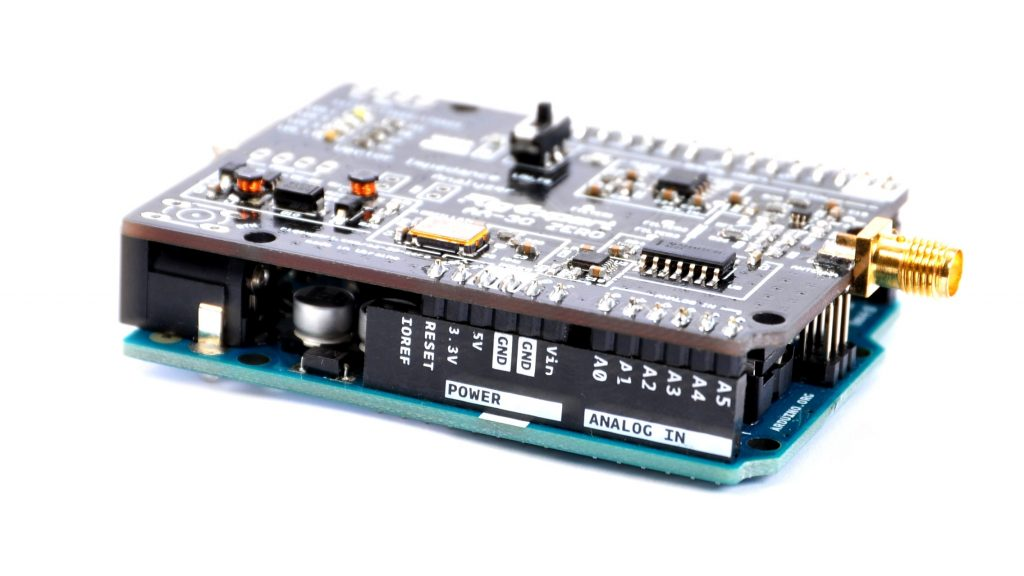# RigExpertZero library for Arduino

20.02.2020DSC_8266-1024x576Dear friends!

We are in a hurry to announce great news for the current and future owners of the AA-30 ZERO analyzer.
Our engineers have developed a special library with program code for Arduino, which will allow enthusiasts to simplify the development of their program code for together work between Arduino and the AA-30.ZERO analyzer.

A bit more about working with the library.

First of all, do not forget to include the library in your program code. This is done using the

`#include "RigExpertZero.h"`

Next, you need to use the RigExpertZero ZERO(RX_PIN, TX_PIN) command to indicate to which Arduino pins the pins of the RX and TX analyzer are connected.
This can be done explicitly:

`RigExpertZero ZERO(4, 7)`

Or using the #define directive:

`#define RX_PIN 4`
`#define TX_PIN 7`
`RigExpertZero ZERO(RX_PIN, TX_PIN);`

Excellent! All necessary configuration procedures are completed.

Let’s consider two main functions of the library.

The `ZERO.startZero()` function initializes the analyzer and prepares it for work. This function sends the VER command to analyzer and expects a correct answer. If the answer is received function returns “true“. If a failure occurs function will return a “false.
This feature can be used to verify the reliability of the analyzer connection.
For example:

`while(!ZERO.startZero()){`
`Serial.print("ZERO not found!\r\n");`
`delay(300);`
`}`
`Serial.print("It is ZERO!\r\n");`

The `ZERO.startMeasure ()`function starts a measurement at a given frequency. The frequency value can be set directly or through a variable.
The ZERO.startMeasure () function also returns “true” if successful and “false” if something went wrong.
The measurement result is stored as an array of data in the memory of Arduino.

To obtain the measurement results that are of direct interest to us, the following functions are used:

`ZERO.getR()` – returns the resistance value in a series system.

`ZERO.getRp()`– returns the resistance value in a parallel system.

`ZERO.getX()`– returns the value of reactance in a series system.

`ZERO.getXp()`– returns the value of reactance in a parallel system.

`ZERO.getSWR()`– returns the value of the standing wave ratio (SWR).

`ZERO.getRL()`– returns the value of return loss.

`ZERO.getZ()`– returns the value of the impedance module.

`ZERO.getPhase()`– returns the phase value of the reflected wave.

`ZERO.getRho()`– returns the value of the reflection coefficient.

It is not difficult to guess the purpose of each function based on its name.

Important! The library works correctly with the analyzer with firmware no lower than version 2.0

You can update the analyzer firmware using the FlashTool utility.

In the examples, you will find a sketch that will take 30 measurements in the frequency range from 1 to 30 MHz and issue the frequency values and the values of all possible parameters to the serial port.

In the future, we plan to release several practical constructs based on this library. Follow the news on our website and group on Facebook.

Respectfully,
RigExpert Crew